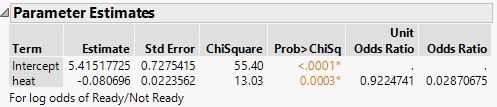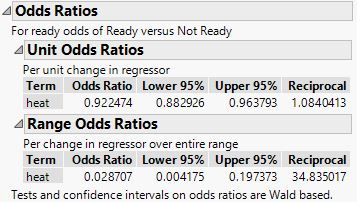Choose Language Hide Translation Bar
Highlighted

## Logistic regression with multiple outcome variables

I am running logistic regression analyses on a dataset where the outcome has multiple categorical variables.  In this case, I am using total number of humans present (continuous variable) to predict the behavior of gibbons (the behaviors are categorical).  I am trying to use the parameter estimates to determine which specific behaviors are affected by the number of humans, but I am having trouble interoperating the results.  I can see the results for each individual behavior, and the Wald test to show whether that behavior is affected by number of people, but the last behavior alphabetically on my list (vocalize) does not output any numbers, so I do not know how to see if this variable is affected.  Am I interoperating these results correctly?  How do I see if the last behavior (vocalize) is affected by number of people?

2 ACCEPTED SOLUTIONS

Accepted Solutions
Highlighted

## Re: Logistic regression with multiple outcome variables

If you have more than two levels in the response variables, then you are using ordinal or nominal logistic regression. In both of these cases, the cumulative logits or generalized logits, respecitvely, use the last level for the odds, so it cannot estimated. So if you have k levels, then there are k-1 logits with their associated parameters.

The best way to interpret these logits is either by odds ratios (relative to the last level) or with a specific visualization, such as the prediction profiler in JMP.

Learn it once, use it forever!
Highlighted

## Re: Logistic regression with multiple outcome variables

I must admit that I do not know the subset of JMP features that are available in the student version, but please try these suggestions and let me know what you find.

1. Using Fit Y by X, you launch a Logistic platform. You already foiund the p-values for the Wald tests to judge significance. Click the red triangle at the top of the window and select Odds Ratios. Two of them appear to the right of the parameter estimates and the p-values. The Unit Odds Ratio is the ratio for 1 unit change in the predictor. The other Odds Ratio is for the change over the whole range (minimum to maximum) of your predictor.

(Note: if you launch Logistic with multiple responses, you will get parallel models in the same window. Try this: press and hold the CTRL on Windows or Command on Macintosh, and then click the red triangle. This way the odds ratios will be computed for all of the responses, instead of asking one response at a time. This trick is called broadcasting and works with most features in JMP!)

2. Alternatively, select Analyze > Fit Model. Assign your responses to the Y role and add your predictor to the effects list. Click the Run button and you should get the same basic analysis as you did from Logistic, but with importance differences. The parameter estimates use likelihood ratio tests instead of Wald tests. Also, click the red triangle at the top and select Profilers > Profiler. It will appear at the bottom of the window. Now the profiler shows you the predicted probabilities of each response level. You can estimate the probabilities under some reference level of the predictor, then estimate the probabilites under other conditions and form any odds ratio that you might need.

There are many more features in the Logistic and the Nominal Logisitic Fit platforms, but I don't want to overwhelm you all at once. Please let me know if this explanation was helpful, or if you have any more difficulty or questions.

Learn it once, use it forever!
25 REPLIES 25
Highlighted

## Re: Logistic regression with multiple outcome variables

If you have more than two levels in the response variables, then you are using ordinal or nominal logistic regression. In both of these cases, the cumulative logits or generalized logits, respecitvely, use the last level for the odds, so it cannot estimated. So if you have k levels, then there are k-1 logits with their associated parameters.

The best way to interpret these logits is either by odds ratios (relative to the last level) or with a specific visualization, such as the prediction profiler in JMP.

Learn it once, use it forever!
Highlighted

## Re: Logistic regression with multiple outcome variables

Thanks for the help.  I have a student license of JMP.  I cannot find the odds ratio test and I cannot figure out how to use the profiler with non-numeric values.  Do you know how I can access these functions?  I just am running a fit y by x analysis, so I also do not know how to indicate I am running a nominal logistic regression.

Highlighted

## Re: Logistic regression with multiple outcome variables

I must admit that I do not know the subset of JMP features that are available in the student version, but please try these suggestions and let me know what you find.

1. Using Fit Y by X, you launch a Logistic platform. You already foiund the p-values for the Wald tests to judge significance. Click the red triangle at the top of the window and select Odds Ratios. Two of them appear to the right of the parameter estimates and the p-values. The Unit Odds Ratio is the ratio for 1 unit change in the predictor. The other Odds Ratio is for the change over the whole range (minimum to maximum) of your predictor.

(Note: if you launch Logistic with multiple responses, you will get parallel models in the same window. Try this: press and hold the CTRL on Windows or Command on Macintosh, and then click the red triangle. This way the odds ratios will be computed for all of the responses, instead of asking one response at a time. This trick is called broadcasting and works with most features in JMP!)

2. Alternatively, select Analyze > Fit Model. Assign your responses to the Y role and add your predictor to the effects list. Click the Run button and you should get the same basic analysis as you did from Logistic, but with importance differences. The parameter estimates use likelihood ratio tests instead of Wald tests. Also, click the red triangle at the top and select Profilers > Profiler. It will appear at the bottom of the window. Now the profiler shows you the predicted probabilities of each response level. You can estimate the probabilities under some reference level of the predictor, then estimate the probabilites under other conditions and form any odds ratio that you might need.

There are many more features in the Logistic and the Nominal Logisitic Fit platforms, but I don't want to overwhelm you all at once. Please let me know if this explanation was helpful, or if you have any more difficulty or questions.

Learn it once, use it forever!
Highlighted

## Re: Logistic regression with multiple outcome variables - Odds ratios in JMP

I am running a multiple multinomial logisitc regression and am unable to get JMP to compute the odds ratios as is shown in tutorials using canned data sets.

Following the above instructions:

When I use fit Y by X (broadcasted or not) the odds ratio option does not come up (see first and second screen shots below).

I can find the profiler, but I am really looking for odds ratios so that I can compare them to ones I already computed by hand (see third screen shot on right).

Has anyone else had this problem?  Were you ever able to get JMP to compute odds ratios for a multiple multinomial logistic?

Highlighted

## Re: Logistic regression with multiple outcome variables - Odds ratios in JMP

The odds ratios are available in both the Logistic platform (starting with Fit Y by X) and the Nominal Logistic Fit platform (starting with Fit Model). Using the tutorial example that you cited above, I get this result using the first platform:I get this result using the second platform:Note that odds ratios are only available for a nominal response.

Learn it once, use it forever!
Highlighted

## Re: Logistic regression with multiple outcome variables - Odds ratios in JMP

I think I know what the problem is, The example that you cited has a binary outcome. It sounds, however, like your data has more than two levels. JMP does not compute the odds ratios in such a case. You can save the probability formulas, though, and compute the odds and then the odds ratios with them.

Learn it once, use it forever!
Highlighted

## Re: Logistic regression with multiple outcome variables - Odds ratios in JMP

Dear Mark,

Thanks for your help!  I certainly wish JMP did this automatically. Maybe in version 14 :)

When I save the prob formulas I get the following columns

Lin [F]      Lin [B]    Prob [F]     Prob [B]    Prob [M]     Most likely outcome

Where F, B, and M are my outcomes and M is the reference category.

Questions:

What are the Lin[ ] columns telling me?

Computing this for binary logisitc regression wouldn't be all that hard, but here I think it is a bit trickier.

How do I

- compute the odds for each individual?

- compute the odds for each outcome category group?

Once I have the odds for each outcome group I simply divide these by the combined odds for the refernce group to get the odds ratios (of F compared to M, for example). Is that correct?

Thanks for sharing your statistical know-how!

Highlighted

## Re: Logistic regression with multiple outcome variables - Odds ratios in JMP

The Lin[X] columns contain the linear predictor for the logit function that you fit. If you examine the formula, you will see the same parameter estimates as were shown in the logistic regression results. You don't need it unless you need the logit!

The linear predictor is, n turn, used to compute the probability of each response level as Prob[X]. (It comes from solving log( p / (1-p) ) = Lin[X] for p.) This probability is most useful for your purpose. Note that if you select Graph > Profiler and select Prob[X], be sure to enable the feature to expand the intermediate formulas, otherwise you will profile against Lin[X] instead of the actual predictor variable.

You can use the Prob[X] columns in new column formulas to compute any odds that you want as Prob[X1] divided by Prob[X2]. Likewise, you can then compute any odds ratio that you want from the odds derived this way.

Learn it once, use it forever!
Highlighted

## Re: Logistic regression with multiple outcome variables - Odds ratios in JMP

I see! Thank you!

When I use the Prob[X] columns to compute odds t(Prob[X1] divided by Prob[X2]) that will give me the odds for that individual, correct?

When I report this in a paper I will need to report the odds for each group and the odds ratio for compared groups (F treated compared to M treated or untreated, for exmaple).

When computing group odds do I simply average the odds for that group?

I realize this is more of a statistics question than for JMP per say, but most programs calculate group odds so I can't find other documentation on how to do it by hand.  Thank you!

Article Labels

There are no labels assigned to this post.